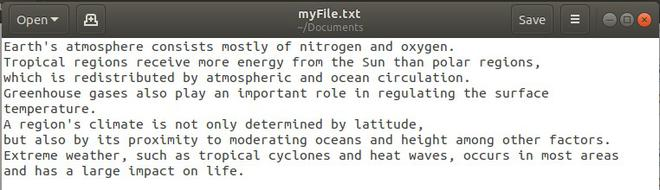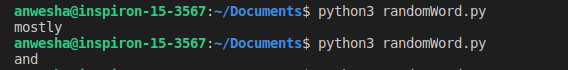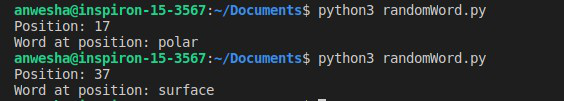GFG App
Open AppBrowser
Continue

# Pulling a random word or string from a line in a text file in Python

File handling in Python is really simple and easy to implement. In order to pull a random word or string from a text file, we will first open the file in read mode and then use the methods in Python’s random module to pick a random word.

There are various ways to perform this operation:

This is the text file we will read from:Method 1: Using random.choice()

Steps:

1. Using with function, open the file in read mode. The with function takes care of closing the file automatically.
2. Read all the text from the file and store in a string
3. Split the string into words separated by space.
4. Use random.choice() to pick a word or string.

## Python

 `# Python code to pick a random ` `# word from a text file ` `import` `random ` ` `  `# Open the file in read mode ` `with ``open``(``"MyFile.txt"``, ``"r"``) as ``file``: ` `    ``allText ``=` `file``.read() ` `    ``words ``=` `list``(``map``(``str``, allText.split())) ` ` `  `    ``# print random string ` `    ``print``(random.choice(words)) `

Note: The split() function, by default, splits by white space. If you want any other delimiter like newline character you can specify that as an argument.

Output:Output for two sample runs

The above can be achieved with just a single line of code like this :

## Python

 `# import required module ` `import` `random ` ` `  `# print random word ` `print``(random.choice(``open``(``"myFile.txt"``,``"r"``).readline().split()))`

Method 2: Using random.randint()

Steps:

1. Open the file in read mode using with function
2. Store all data from the file in a string and split the string into words.
3. Count the total number of words.
4. Use random.randint() to generate a random number between 0 and the word_count.
5. Print the word at that position.

## Python

 `# using randint() ` `import` `random ` ` `  `# open file ` `with ``open``(``"myFile.txt"``, ``"r"``) as ``file``: ` `    ``data ``=` `file``.read() ` `    ``words ``=` `data.split() ` `     `  `    ``# Generating a random number for word position ` `    ``word_pos ``=` `random.randint(``0``, ``len``(words)``-``1``) ` `    ``print``(``"Position:"``, word_pos) ` `    ``print``(``"Word at position:"``, words[word_pos]) `

Output:Output for two sample runs

My Personal Notes arrow_drop_up